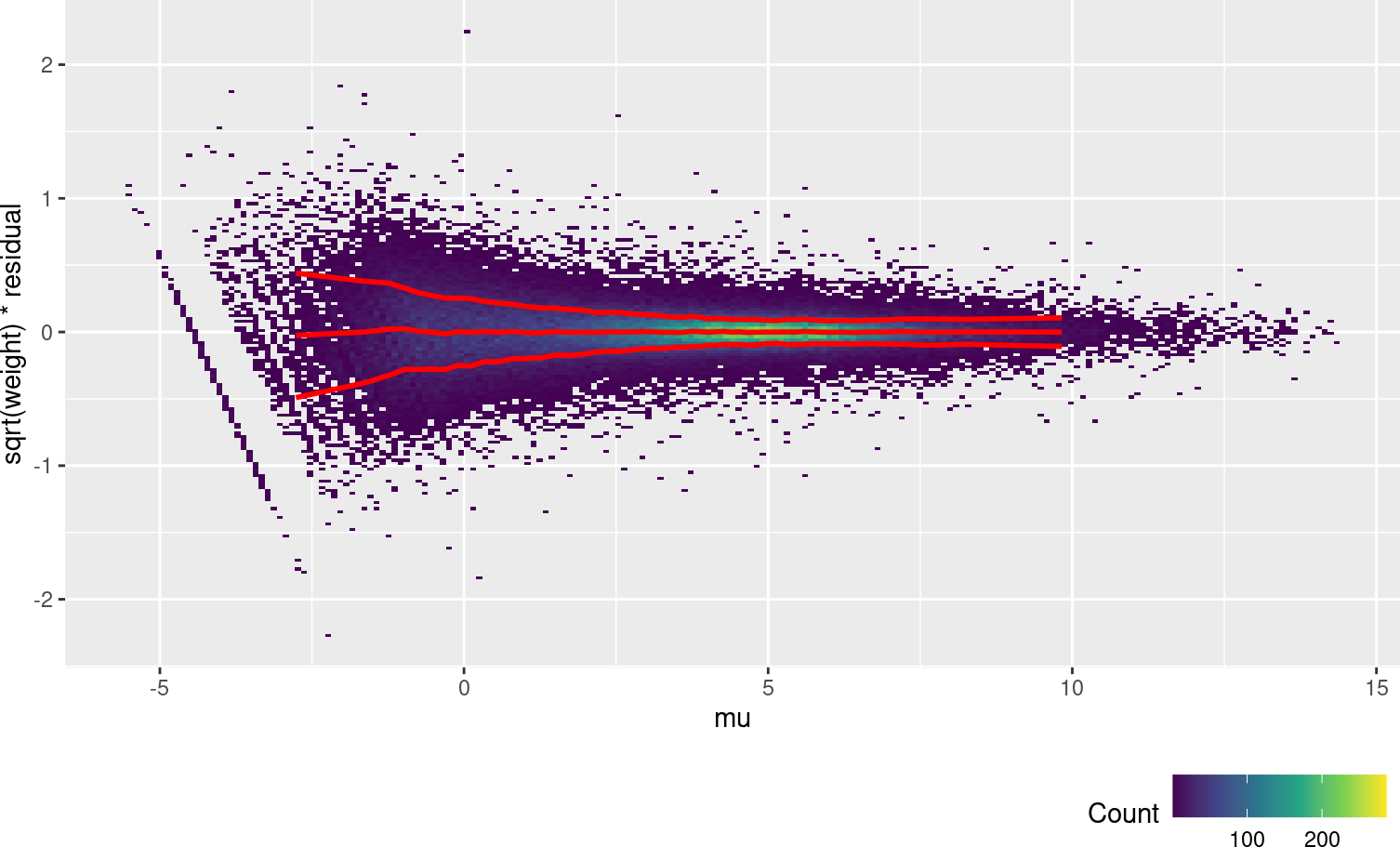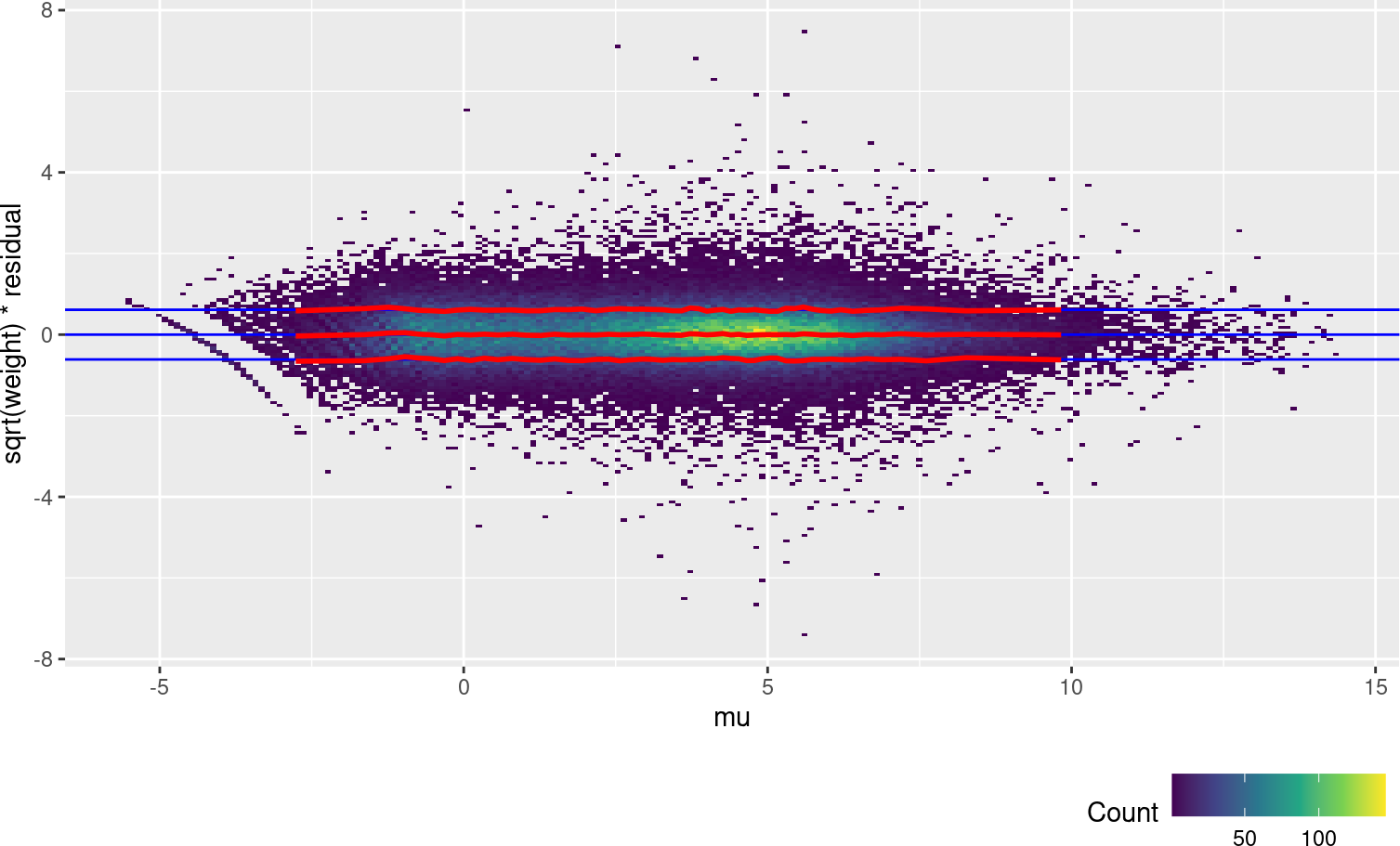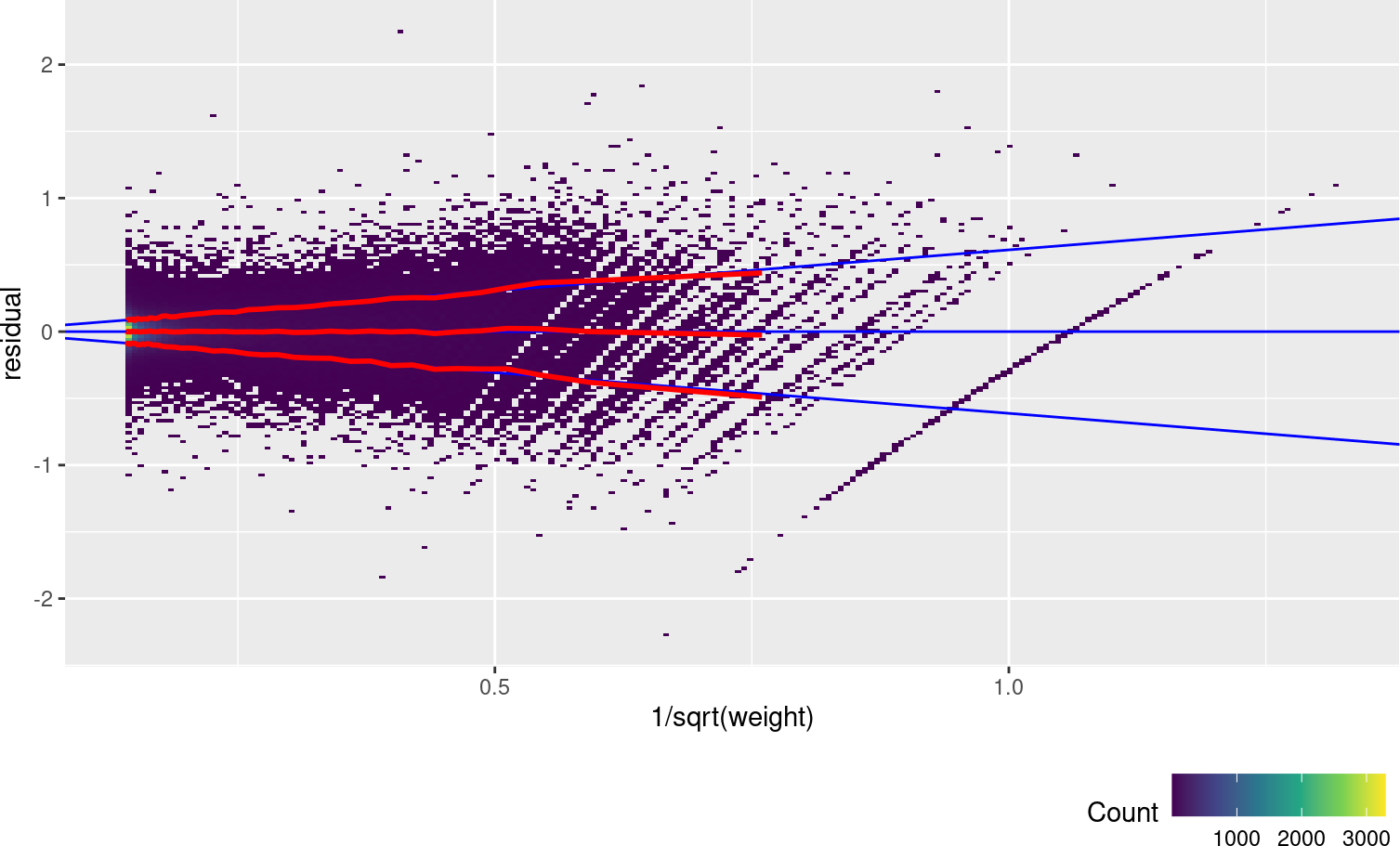# Contents

Let’s look at the airway dataset as an example of a typical small-scale RNA-Seq experiment. In this experiment, four Airway Smooth Muscle (ASM) cell lines are treated with the asthma medication dexamethasone.

The function `weitrix_calibrate_all` will be used to assign precision weights to log Counts Per Million values.

``````library(weitrix)
library(ComplexHeatmap)
library(EnsDb.Hsapiens.v86)
library(edgeR)
library(limma)
library(reshape2)
library(tidyverse)
library(airway)

set.seed(1234)

# BiocParallel supports multiple backends.
# If the default hangs or errors, try others.
# The most reliable backed is to use serial processing
BiocParallel::register( BiocParallel::SerialParam() )``````
``````data("airway")
airway``````
``````## class: RangedSummarizedExperiment
## dim: 63677 8
## assays(1): counts
## rownames(63677): ENSG00000000003 ENSG00000000005 ... ENSG00000273492
##   ENSG00000273493
## rowData names(10): gene_id gene_name ... seq_coord_system symbol
## colnames(8): SRR1039508 SRR1039509 ... SRR1039520 SRR1039521
## colData names(9): SampleName cell ... Sample BioSample``````

# 1 Initial processing

Initial steps are the same as for a differential expression analysis.

``````counts <- assay(airway,"counts")

design <- model.matrix(~ dex + cell, data=colData(airway))

good <- filterByExpr(counts, design=design)
table(good)``````
``````## good
## FALSE  TRUE
## 46817 16860``````
``````airway_dgelist <- DGEList(counts[good,]) %>% calcNormFactors()

airway_lcpm <- cpm(airway_dgelist, log=TRUE, prior.count=1)``````

log2 CPM values have been calculated, with an added “prior” count of (on average) 1 read, so that zeros aren’t sent to negative infinity.

# 2 Conversion to weitrix

``````airway_weitrix <- as_weitrix(airway_lcpm)

# Include row and column information
colData(airway_weitrix) <- colData(airway)
rowData(airway_weitrix) <-
mcols(genes(EnsDb.Hsapiens.v86))[rownames(airway_weitrix),c("gene_name","gene_biotype")]

airway_weitrix``````
``````## class: SummarizedExperiment
## dim: 16860 8
## assays(2): x weights
## rownames(16860): ENSG00000000003 ENSG00000000419 ... ENSG00000273487
##   ENSG00000273488
## rowData names(2): gene_name gene_biotype
## colnames(8): SRR1039508 SRR1039509 ... SRR1039520 SRR1039521
## colData names(9): SampleName cell ... Sample BioSample``````

# 3 Calibration

To calibrate, we need predicted expression levels so we can calculate residuals. The function `weitrix_components` can provide linear model fits to each gene. (We will see a more advanced use of this function later.)

``fit <- weitrix_components(airway_weitrix, design=design)``

Currently the weitrix has weights uniformly equal to 1. Examining residuals, we see a variance trend relative to the linear model prediction.

Each dot in the plot below is a residual weighted by sqrt(weight). The x-axis is the linear model prediction. The y-axis is the weighted residual (all weights are currently 1). The red lines show the mean and the mean +/- one standard deviation.

``weitrix_calplot(airway_weitrix, fit, covar=mu, guides=FALSE)``We will use the function `weitrix_calibrate_all` to set the weights by fitting a gamma GLM with log link function to the weighted squared residuals. 1 over the predictions from this GLM are used as weights. Here we fit a natural spline based on the linear model predictions from `fit`, which are referred to in the formula as `mu`. `well_knotted_spline` is a wrapper around `splines::ns` for natural splines with improved choice of knot locations.

``````airway_cal <- weitrix_calibrate_all(
airway_weitrix,
design = fit,
trend_formula = ~well_knotted_spline(mu,5))``````
``## mu range -5.552401 14.303375 knots -0.8637096  1.4763752  3.6914433  5.6106759  8.0243479``
``metadata(airway_cal)``
``````## \$weitrix
## \$weitrix\$x_name
##  "x"
##
## \$weitrix\$weights_name
##  "weights"
##
## \$weitrix\$all_coef
##                 (Intercept) well_knotted_spline(mu, 5)1
##                  -0.4307917                  -3.0128598
## well_knotted_spline(mu, 5)2 well_knotted_spline(mu, 5)3
##                  -4.0044737                  -4.6128293
## well_knotted_spline(mu, 5)4 well_knotted_spline(mu, 5)5
##                  -3.3897229                  -5.2701723
## well_knotted_spline(mu, 5)6
##                  -3.1086780``````
``weitrix_calplot(airway_cal, fit, covar=mu)``The trend lines (red) for the calibrated weitrix are now uniformly close to 1, 0, -1 (guide lines shown in blue). Weights can now be treated as inverse residual variance.

Another way to check this is to plot the unweighted residuals against 1/sqrt(weight), i.e. the residual standard deviation.

``weitrix_calplot(airway_cal, fit, funnel=TRUE)``We can also check each sample individually.

``weitrix_calplot(airway_cal, fit, covar=mu, cat=col)``